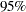Previous Page | Next Page

 The REG Procedure
 Parameter Estimates and Associated Statistics

The following example uses the fitness data from Example 74.2. Figure 74.30 shows the parameter estimates and the tables from the SS1, SS2, STB, CLB, COVB, and CORRB options:

```proc reg data=fitness;
model Oxygen=RunTime Age Weight RunPulse MaxPulse RestPulse
/ ss1 ss2 stb clb covb corrb;
run;
```

The procedure first displays an analysis of variance table (Figure 74.29). Thestatistic for the overall model is significant, indicating that the model explains a significant portion of the variation in the data.

Figure 74.29 ANOVA Table
The REG Procedure
Model: MODEL1
Dependent Variable: Oxygen

Analysis of Variance
Source DF Sum of
Squares
Mean
Square
F Value Pr > F
Model 6 722.54361 120.42393 22.43 <.0001
Error 24 128.83794 5.36825
Corrected Total 30 851.38154

 Root MSE R-Square 2.31695 0.8487 47.3758 0.8108 4.89057

The procedure next displays parameter estimates and some associated statistics (Figure 74.30). First, the estimates are shown, followed by their standard errors. The next two columns of the table contain thestatistics and the corresponding probabilities for testing the null hypothesis that the parameter is not significantly different from zero. These probabilities are usually referred to as-values. For example, the Intercept term in the model is estimated to be 102.9 and is significantly different from zero. The next two columns of the table are the result of requesting the SS1 and SS2 options, and they show sequential and partial sums of squares (SS) associated with each variable. The standardized estimates (produced by the STB option) are the parameter estimates that result when all variables are standardized to a mean of 0 and a variance of 1. These estimates are computed by multiplying the original estimates by the standard deviation of the regressor (independent) variable and then dividing by the standard deviation of the dependent variable. The CLB option adds the upper and lowerconfidence limits for the parameter estimates; thelevel can be changed by specifying the ALPHA= option in the PROC REG or MODEL statement.

Figure 74.30 SS1, SS2, STB, CLB, COVB, and CORRB Options: Parameter Estimates
Parameter Estimates
Variable DF Parameter
Estimate
Standard
Error
t Value Pr > |t| Type I SS Type II SS Standardized
Estimate
95% Confidence Limits
Intercept 1 102.93448 12.40326 8.30 <.0001 69578 369.72831 0 77.33541 128.53355
RunTime 1 -2.62865 0.38456 -6.84 <.0001 632.90010 250.82210 -0.68460 -3.42235 -1.83496
Age 1 -0.22697 0.09984 -2.27 0.0322 17.76563 27.74577 -0.22204 -0.43303 -0.02092
Weight 1 -0.07418 0.05459 -1.36 0.1869 5.60522 9.91059 -0.11597 -0.18685 0.03850
RunPulse 1 -0.36963 0.11985 -3.08 0.0051 38.87574 51.05806 -0.71133 -0.61699 -0.12226
MaxPulse 1 0.30322 0.13650 2.22 0.0360 26.82640 26.49142 0.52161 0.02150 0.58493
RestPulse 1 -0.02153 0.06605 -0.33 0.7473 0.57051 0.57051 -0.03080 -0.15786 0.11480

The final two tables are produced as a result of requesting the COVB and CORRB options (Figure 74.31). These tables show the estimated covariance matrix of the parameter estimates, and the estimated correlation matrix of the estimates.

Figure 74.31 SS1, SS2, STB, CLB, COVB, and CORRB Options: Covariances and Correlations
Covariance of Estimates
Variable Intercept RunTime Age Weight RunPulse MaxPulse RestPulse
Intercept 153.84081152 0.7678373769 -0.902049478 -0.178237818 0.280796516 -0.832761667 -0.147954715
RunTime 0.7678373769 0.1478880839 -0.014191688 -0.004417672 -0.009047784 0.0046249498 -0.010915224
Age -0.902049478 -0.014191688 0.009967521 0.0010219105 -0.001203914 0.0035823843 0.0014897532
Weight -0.178237818 -0.004417672 0.0010219105 0.0029804131 0.0009644683 -0.001372241 0.0003799295
RunPulse 0.280796516 -0.009047784 -0.001203914 0.0009644683 0.0143647273 -0.014952457 -0.000764507
MaxPulse -0.832761667 0.0046249498 0.0035823843 -0.001372241 -0.014952457 0.0186309364 0.0003425724
RestPulse -0.147954715 -0.010915224 0.0014897532 0.0003799295 -0.000764507 0.0003425724 0.0043631674

Correlation of Estimates
Variable Intercept RunTime Age Weight RunPulse MaxPulse RestPulse
Intercept 1.0000 0.1610 -0.7285 -0.2632 0.1889 -0.4919 -0.1806
RunTime 0.1610 1.0000 -0.3696 -0.2104 -0.1963 0.0881 -0.4297
Age -0.7285 -0.3696 1.0000 0.1875 -0.1006 0.2629 0.2259
Weight -0.2632 -0.2104 0.1875 1.0000 0.1474 -0.1842 0.1054
RunPulse 0.1889 -0.1963 -0.1006 0.1474 1.0000 -0.9140 -0.0966
MaxPulse -0.4919 0.0881 0.2629 -0.1842 -0.9140 1.0000 0.0380
RestPulse -0.1806 -0.4297 0.2259 0.1054 -0.0966 0.0380 1.0000

For further discussion of the parameters and statistics, see the section Displayed Output, and Chapter 4, Introduction to Regression Procedures.Previous Page | Next Page | Top of Page